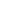# Represent two-digit sums and differences involving length by using the ruler as a number line

EngageNY 60 min(s)Support for English Language Learners
In this lesson, students represent two-digit sums and differences on a number line, and write a number sentence to represent the addition or subtraction situation. Students show how they solve these problems on the number line with different starting points, and they consider how two different measurement situations can result in the same total and are thus equal to each other (e.g., 25 + 11 = 15 + 15 + 6).